# Catalogue Entry: NATP00221

## Newton's Waste Book (Part 2)

Source: MS Add. 4004, ff. 15v-50r, Cambridge University Library, Cambridge, UK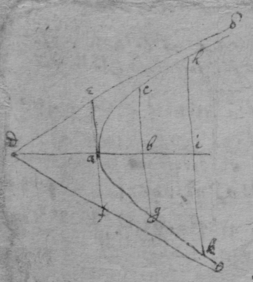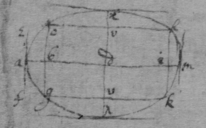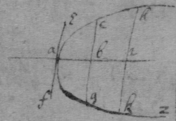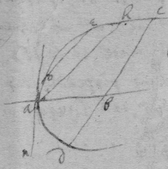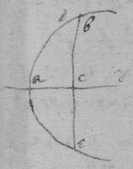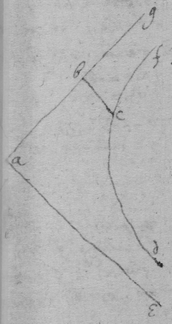October 1664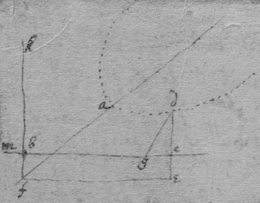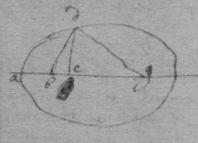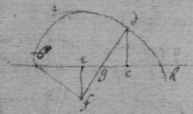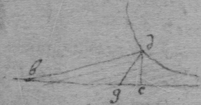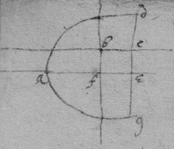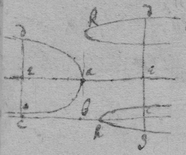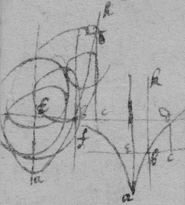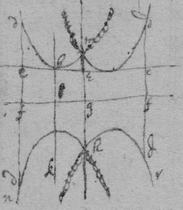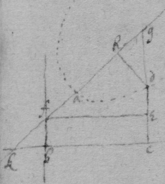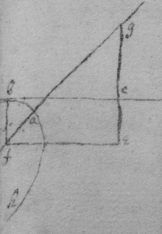For the first equation of the first sort

 For the 2d

 For the 3d

 For the 4th

 For the 5t

 For the 6t &c

 For the first Equation of the seacond Sort

 For the seacond

 For the 3d.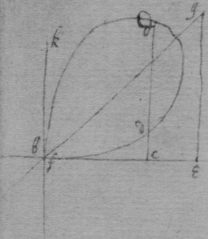November 1664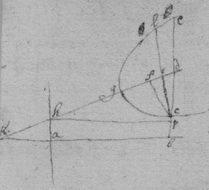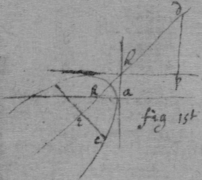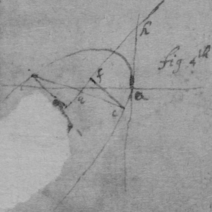November 1664

 A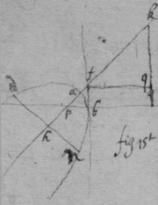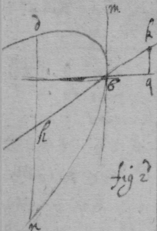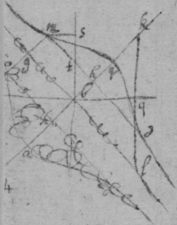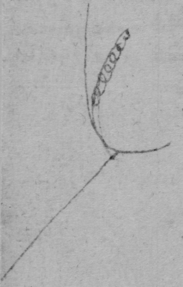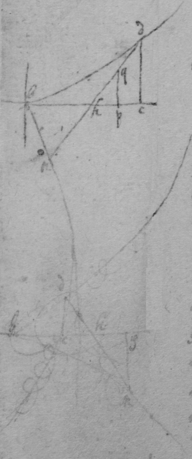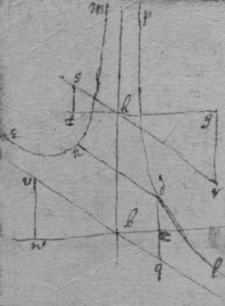B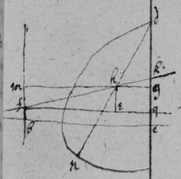This line is a streight one the equation being divisible by $b=y=0$

 Endeavor not to find the quantity d in these cases, but suppose it given[Editorial Note 1]

[Editorial Note 1] There is a line connecting the end of this note to the following one

 Or else C

 December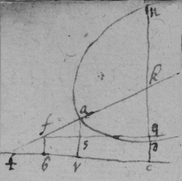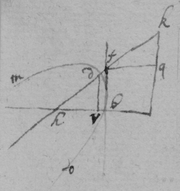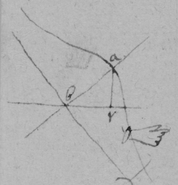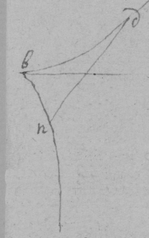F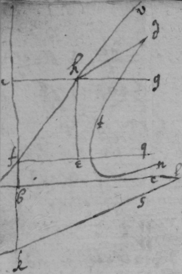G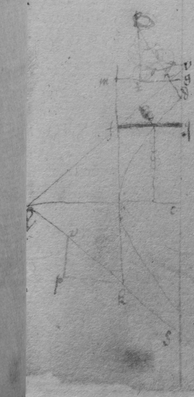December 1664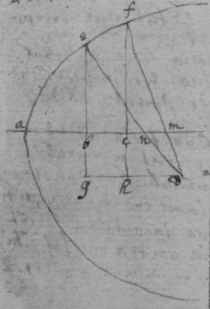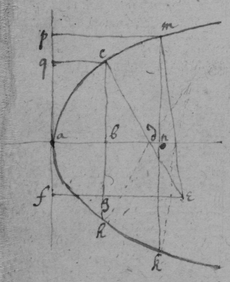TheoremaDecember 1664.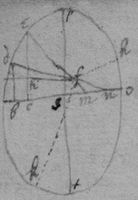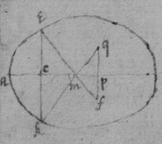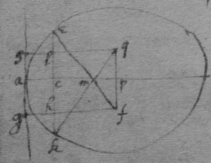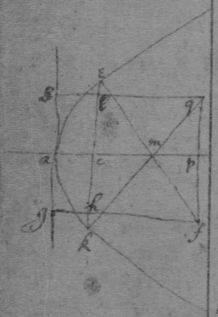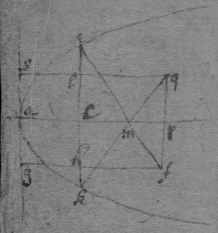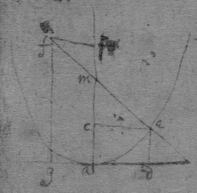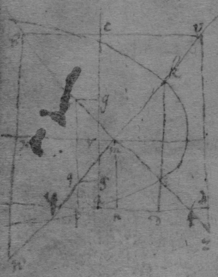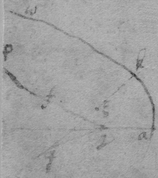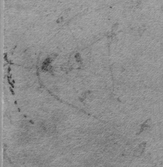Feb 1664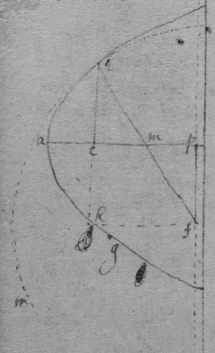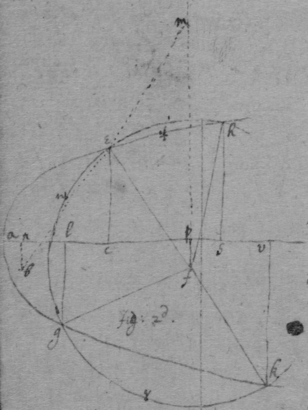Another way.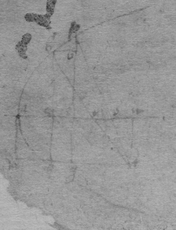December 1664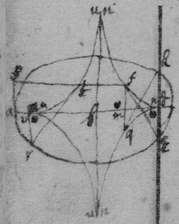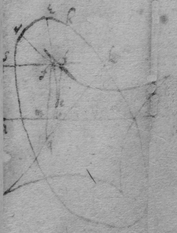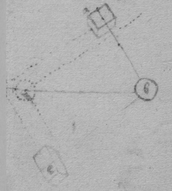Of compound force.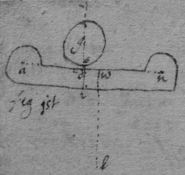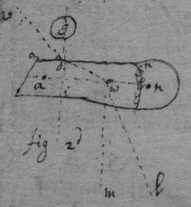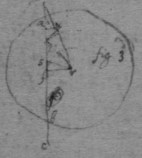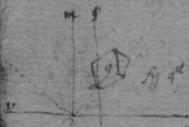May 20th 1665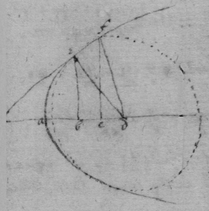Observation 1st

 Observacion 2d

 An universall theorem for tangents to crooked lines, when $y\perp x$.

 See Des Cartes his Geometry. booke 2d, pag 42, 46, 47. Or thus, $\begin{array}{ccccccccc}{x}^{3}& -& bxx& -& cdx& +& dyx& +& bcd\\ 2& & 1& & 0& & 0& & -1& \end{array}$ { $\frac{2xxy-bxy}{-dx}\phantom{\rule{0.5em}{0ex}}\frac{+bcdy}{dxx}=v$ }. And $\frac{bcy}{xx}+\frac{by}{d}-\frac{2xy}{d}=v$ .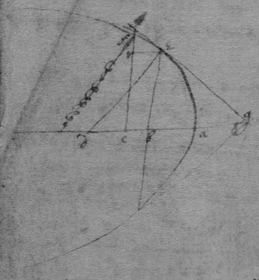An universall theorem for drawing tangents to crooked lines when x & y intersect at any determined angle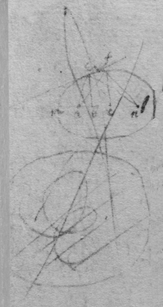A=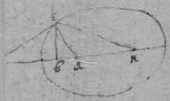[Editorial Note 2] The rest of the page is damaged.